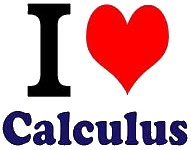## Τετάρτη 14 Σεπτεμβρίου 2022

### Exercises in Calculus by Norman Dobson

These exercises were given by the late Norman Dobson under the title "Final Exam Problems". They are excellent problems in preparation for examinations.

###Calculus I NYA - Contents

Limits (ps, pdf)
Continuity (ps, pdf)
Definition of Derivative (ps, pdf)
Differentiation u(ps, pdf)
Tangents and Normals (ps, pdf)
Related Rates (ps, pdf)
Higher Derivatives (ps, pdf)
Curve Sketching (ps, pdf)
Optimization (ps, pdf)
Integration (ps, pdf)
Differential Equations (ps, pdf)
Area (ps, pdf)
Various (ps, pdf)

### Calculus II NYB - Contents

Integration (ps, pdf)
Limits (ps, pdf)
Volumes of Revolution (ps, pdf)
Sequences, Geometric and Telescoping Series (ps, pdf)
Positive Term Series (ps, pdf)
Alternating Series (ps, pdf)

#### Δημοσίευση σχολίου